Courses

## 8 Questions MCQ Test Question Bank for GATE Computer Science Engineering | ER-Model (Advance Level)- 1

Description
This mock test of ER-Model (Advance Level)- 1 for Computer Science Engineering (CSE) helps you for every Computer Science Engineering (CSE) entrance exam. This contains 8 Multiple Choice Questions for Computer Science Engineering (CSE) ER-Model (Advance Level)- 1 (mcq) to study with solutions a complete question bank. The solved questions answers in this ER-Model (Advance Level)- 1 quiz give you a good mix of easy questions and tough questions. Computer Science Engineering (CSE) students definitely take this ER-Model (Advance Level)- 1 exercise for a better result in the exam. You can find other ER-Model (Advance Level)- 1 extra questions, long questions & short questions for Computer Science Engineering (CSE) on EduRev as well by searching above.
QUESTION: 1

### Student and courses enrolled, is an example of

Solution:

Many students can be enrolled in one or more courses and one course may have one or more students hence relation is many to many.

QUESTION: 2

### A primary key if combined with a foreign key creates

Solution:

A foreign key is a key of a relation which is primary key of some other relation. A primary key is combined with foreign key then are ferential constraint is set up between the two relation, hence it creates a parent-child relationship between the tables that connects them.

QUESTION: 3

### It is desired to design an object-oriented employee record system for a company. Each employee has a name, unique id and salary. Employees belong to different categories and their salary is determined by their category. The functions get Name, get ld and compute Salary are required. Given the class hierarchy below, possible locations for these functions are: 1. getld is implemented in the superclass. 2. getId is implemented in the subclass. 3. getName is an abstract function in the superclass. 4. getName is implemented in the superclass. 5. getName is implemented in the subclass: 6. getSalary is an abstract function in the superclass. 7. getSalary is implemented in the superclass. 8. getSalary is implemented in the subclass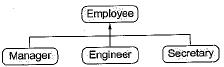Choose the best design

Solution:

Each employee has a unique id, name and salary, Employees may have different salary as per their category e.g. Manager, Engineer or Secretary.
Id is unique for each employee for a given employee with Id name is unique.
Hence, get Id and get name must be implemented in Superclass as whatever their designation it will not change. Get salary must be an abstract function in superclass and implemented in subclasses.

QUESTION: 4

Let E1 and E2 be two in an E/R diagram with simple single-valued attributes R1, and R2 are two relationship between E1, and E2, where R1, is one-to many and R2 is many-to-many. R1, and R2 do not have any attributes of their own. What is the minimum number of tables required to represent this situation in the relational model?

Solution:

The given Relations R1 and R2 can be drawn as: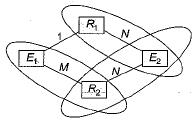For relations R1, and R2, 3 tables are required as circled above.

QUESTION: 5

Consider the following ER diagram: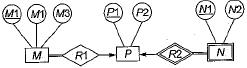The minimum number of table needed to represent M, N, P, R1, R2 is.

Solution:

Minimum number of tables needed is 2 as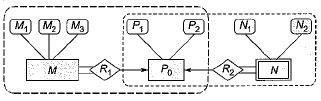1st table have the relation between M and Pand 2nd table as P and N.

QUESTION: 6

Consider the following ER diagram:Which of the following is a correct attribute set for one of the tables for the correct answer to the above question?

Solution:

The tables needed for the given ER-diagram are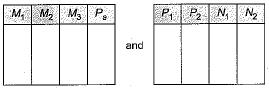which is covering aIl the attributes.

QUESTION: 7

Consider the following entity relationship diagram (ERD), where two entities E1 and E2 have a relation R of cardinality 1 : m.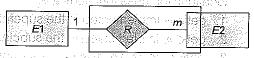The attributes of E1 are A11, A12 and A13where , A11 is key attribute. The attributes of E2 are A21, A22, and A23 where A21 is the key attribute and A23 is a multi-valued attribute. Relation R does not have any attribute. A relational database containing minimum number of tables with each table satisfying the requirements of the third normal form (3NF) is designed from the above ERD. The number of tables in the database is

Solution: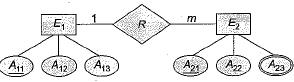Since each table is to in 3rd normal form for each multi-valued attribute individual table is to be created. Hence, total number of tables in the- database is 3.
1 for entity set E1, 2 for entity set E2 and 3 for attributes A23.

QUESTION: 8

​Consider the relation Student (name, sex, marks), where the primary key is shown underlined, pertaining to students in a class that has at least ( One boy and one girl. What does the following relational algebra expression produce? [Note: p is the rename, operator]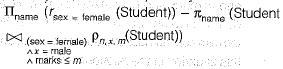Solution:

The given expression can be understood as: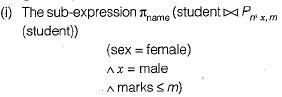gives the name of female students who have less marks than other boys.
(ii) The expression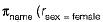(student)) gives the name of all the female student from (i) and
(iii) The given expression finally calculates the names of female student who have marks more than all the boys in the class.